## How to Calculate and Solve for Relative Apparent Viscosity (for Concentrated suspension) | Rheology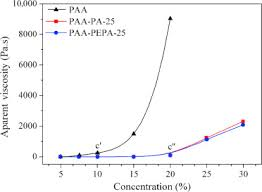The image above represents relative apparent viscosity (for concentrated suspension).

To compute for relative apparent viscosity (for concentrated suspension), three essential parameters are needed and these parameters are Intrinsic Viscosity (ηi), Solid Landing (φ) and Maximum Solid Landing (φm).

The formula for calculating relative apparent viscosity (for concentrated suspension):

ηra = (1 – φ / φm)-(ηim

Where:

ηra = Relative Apparent Viscosity
ηi = Intrinsic Viscosity
φ = Solid Landing
φm = Maximum Solid Landing

Let’s solve an example;
Find the relative apparent viscosity when the intrinsic viscosity is 20, the solid landing is 32 and the maximum solid landing is 12.

This implies that;

ηi = Intrinsic Viscosity = 20
φ = Solid Landing = 32
φm = Maximum Solid Landing = 12

ηra = (1 – φ / φm)-(ηim
ηra = (1 – 32 / 12)-(20) x 12
ηra = (1 – 2.66)-240
ηra = (-1.66)-240
ηra = 5.705e-54

Therefore, the relative apparent viscosity (for concentrated suspension) is 5.705e-54.

## How to Calculate and Solve for Sedimentation of Concentrated Suspension | Rheology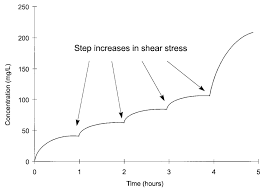The image above represents sedimentation of concentrated suspension.

To compute for sedimentation of concentrated suspension, five essential parameters are needed and these parameters are Change in Density between the Dispersed and Continuous Phase of the Suspension (ΔP), Acceleration due to Gravity (g), Particle Radius (a), Viscosity of Continuous Phase (η) and Solid Landing (φ).

The formula for calculating sedimentation of concentrated suspension:

v = 2ΔPga2 / (1 – φ)5 ± 0.25

Where:

v = Sedimentation of Concentrated Suspension
ΔP = Change in Density between the Dispersed and Continuous Phase of the Suspension
g = Acceleration due to Gravity
η = Viscosity of Continuous Phase
φ = Solid Landing

Let’s solve an example;
Find the sedimentation of concentrated suspension when the change in density between the dispersed and continuous phase of the suspension is 20, the acceleration due to gravity is 12, the particle radius is 32, the viscosity of continuous phase is 14 and the solid landing is 0.

This implies that;

ΔP = Change in Density between the Dispersed and Continuous Phase of the Suspension = 20
g = Acceleration due to Gravity = 12
a = Particle Radius = 32
η = Viscosity of Continuous Phase = 14
φ = Solid Landing = 0

v = 2ΔPga2 / (1 – φ)5 ± 0.25
v = 2 x 20 x 12 x 322 / 9 x 14 x (1 – 0)5 + 0.25
v = 2 x 20 x 12 x 1024 / 126 x (1)5.25
v = 491520 / 126 x 1
v = 3900.95 x 1
v = 3900.95

Therefore, the sedimentation of concentrated suspension is 3900.95.

## How to Calculate and Solve for Sedimentation of Dilute Suspension | Rheology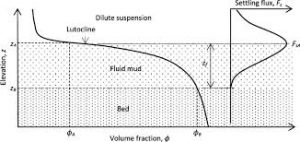The image above represents sedimentation of dilute suspension.

To compute for sedimentation of dilute suspension, four essential parameters are needed and these parameters are Change in Density between the Dispersed and Continuous Phase of the Suspension (ΔP), Acceleration due to Gravity (g), Particle Radius (a) and Viscosity of Continuous Phase (η).

The formula for calculating sedimentation of dilute suspension:

v = 2ΔPga2 /

Where:

v = Sedimentation of Dilute Suspension
ΔP = Change in Density between the Dispersed and Continuous Phase of the Suspension
g = Acceleration due to Gravity
η = Viscosity of Continuous Phase

Let’s solve an example;
Find the sedimentation of dilute suspension when the change in density between the dispersed and continuous phase of the suspension is 10, the acceleration due to gravity is 9.8, particle radius is 22 and the viscosity of continuous phase is 11.

This implies that;

ΔP = Change in Density between the Dispersed and Continuous Phase of the Suspension = 10
g = Acceleration due to Gravity = 9.8
a = Particle Radius = 22
η = Viscosity of Continuous Phase = 11

v = 2ΔPga2 /
v = 2 x 10 x 9.8 x 222 / 9 x 11
v = 2 x 10 x 9.8 x 484 / 99
v = 94864 / 99
v = 958.2

Therefore, the sedimentation of dilute suspension is 958.2.

## How to Calculate and Solve for Einsten Relative Apparent Viscosity (for Dilute System or Suspension) | Rheology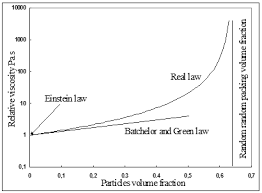The image above represents einstein relative apparent viscosity.

To compute for einstein relative apparent viscosity, one essential parameter is needed and these parameter is Solid Landing (φ).

The formula for calculating einstein relative apparent viscosity:

ηra = 1 + 25φ

Where:

ηra = Einstein Relative Apparent Viscosity
φ = Solid Landing

Let’s solve an example;
Find the einstein relative apparent viscosity when the solid landing is 24.

This implies that;

φ = Solid Landing = 24

ηra = 1 + 25φ
ηra = 1 + 25 x 24
ηra = 1 + 600
ηra = 601

Therefore, the einstein relative apparent viscosity is 601.

## How to Calculate and Solve for Relative Apparent Viscosity | Rheology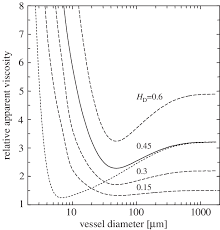The image above represents relative apparent viscosity.

To compute for relative apparent viscosity, two essential parameters are needed and these parameters are Viscosity of Non-Newtonian Fluid (ηa) and Viscosity of Continuous Phase (ηc).

The formula for calculating relative apparent viscosity:

ηra = ηa / ηc

Where:

ηra = Relative Apparent Viscosity
ηa = Viscosity of Non-Newtonian Fluid
ηc = Viscosity of Continuous Phase

Let’s solve an example;
Find the relative apparent viscosity when the viscosity of non-newtonian fluids is 44 and the viscosity of continuous phase is 11.

This implies that;

ηa = Viscosity of Non-Newtonian Fluid = 44
ηc = Viscosity of Continuous Phase = 11

ηra = ηa / ηc
ηra = 44 / 11
ηra = 4

Therefore, the relative apparent viscosity is 4.

Calculating for Viscosity of Non-Newtonian Fluids when the Relative Apparent Viscosity and the Viscosity of Continuous Phase is Given.

ηa = ηra x ηc

Where;

ηa = Viscosity of Non-Newtonian Fluid
ηra = Relative Apparent Viscosity
ηc = Viscosity of Continuous Phase

Let’s solve an example;
Find the viscosity of non-newtonian fluid when the relative apparent viscosity is 16 and the viscosity of continuous phase is 8.

This implies that;

ηra = Relative Apparent Viscosity = 16
ηc = Viscosity of Continuous Phase = 8

ηa = ηra x ηc
ηa = 16 x 8
ηa = 128

Therefore, the viscosity of non-newtonian fluid is 128.

## How to Calculate and Solve for Viscosity of Bingham Fluids | Rheology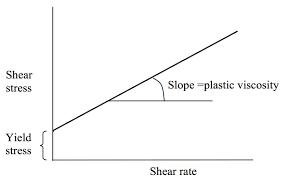The image above represents viscosity of bingham fluids.

To compute for viscosity of bingham fluids, three essential parameters are needed and these parameters are Yield Stress (ζy), Shear Rate (γ) and Co-efficient of Rigidity (ηB).

The formula for calculating viscosity of bingham fluids:

ηa = ηB + ζy / γ

Where:

ηa = Viscosity of Bingham Fluids
ζy = Yield Stress
γ = Shear Rate
ηB = Co-efficient of Rigidity

Let’s solve an example;
Find the viscosity of bingham fluids when the yield stress is 21, the shear rate is 18 and co-efficient of rigidity is 14.

This implies that;

ζy = Yield Stress = 21
γ = Shear Rate = 18
ηB = Co-efficient of Rigidity = 14

ηa = ηB + ζy / γ
ηa = 14 + 21 / 18
ηa = 14 + 1.166
ηa = 15.16

Therefore, the viscosity of bingham fluids is 15.16.

Calculating for Yield Stress when the Viscosity of Bingham Fluids, the Shear Rate and the Co-efficient of Rigidity is Given.

ζy = (ηa – ηB) γ

Where;

ζy = Yield Stress
ηa = Viscosity of Bingham Fluids
γ = Shear Rate
ηB = Co-efficient of Rigidity

Let’s solve an example;
Find the yield stress when the viscosity of bingham fluids is 12, the shear rate is 14 and the co-efficient of rigidity is 8.

This implies that;

ηa = Viscosity of Bingham Fluids = 12
γ = Shear Rate = 14
ηB = Co-efficient of Rigidity = 8

ζy = (ηa – ηB) γ
ζy = (12 – 8) 14
ζy = (4) 14
ζy = 56

Therefore, the yield stress is 56.

## How to Calculate and Solve for Viscosity of Pseudoplastic Fluids | Rheology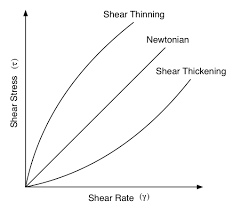The image above represents viscosity of pseudoplastic fluids.

To compute for viscosity of pseudoplastic fluids, three essential parameters are needed and these parameters are Flow Index (n), Shear Rate (γ) and Consistency (ηp).

The formula for calculating viscosity of pseudoplastic fluids:

ηo = ηp γn – 1

Where:

ηo = Viscosity of Pseudoplastics Fluids
n = Flow Index
γ = Shear Rate
ηp = Consistency

Let’s solve an example;
Find the viscosity of pseudoplastic fluids when the flow index is 20, the shear rate is 14 and the consistency is 10.

This implies that;

n = Flow Index = 20
γ = Shear Rate = 14
ηp = Consistency = 10

ηo = ηp γn – 1
ηo = 10 x 1420 – 1
ηo = 10 x 1419
ηo = 10 x 5.97e+21
ηo = 5.97

Therefore, the viscosity of pseudoplastic fluids is 5.97e+22.

## How to Calculate and Solve for Viscosity of Newtonian Fluids | Rheology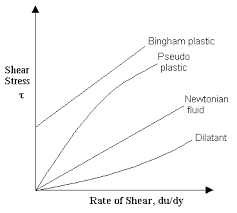The image above represents viscosity of newtonian fluids.

To compute for viscosity of newtonian fluids, two essential parameters are needed and these parameters are Shear Stress (ζ) and Shear Rate (γ).

The formula for calculating viscosity of newtonian fluids:

η = ζ / γ

Where:

η = Viscosity of Newtonian Fluids
ζ = Shear Stress
γ = Shear Rate

Let’s solve an example;
Find the viscosity of newtonian fluids when the shear stress is 15 and the shear rate is 5.

This implies that;

ζ = Shear Stress = 15
γ = Shear Rate = 5

η = ζ / γ
η = 15 / 5
η = 3

Therefore, the viscosity of newtonian fluids is 3.

Calculating for Shear Stress when the Newtonian Fluids and the Shear Rate is Given.

ζ = η x γ

Where;

ζ = Shear Stress
η = Viscosity of Newtonian Fluids
γ = Shear Rate

Let’s solve an example;
Find the shear stress when the newtonian fluids is 20 and the shear rate is 4.

This implies that;

η = Viscosity of Newtonian Fluids = 20
γ = Shear Rate = 4

ζ = η x γ
ζ = 20 x 4
ζ = 80

Therefore, the shear stress is 80.